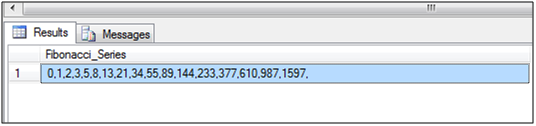# 1.     Background

The purpose of this article is to describe how to generate Fibonacci series in SQL Server. As per the definition of Fibonacci series, first two numbers are 0 and 1 and each subsequent number is the sum of previous two numbers in the series.

Fibonacci series: 0,1,2,3,5,8,13,21,34,55,89,144,233,377,610,987,1597.

Following are the places where we can utilize Fibonacci series:

·         MERGE sort
·         Search Algorithms
·         Network Topology
·         Lossy compression in Data encoding, Streaming Media, and internet telephony.

# 2. Script to generate Fibonacci Series in SQL Server: We are usingWHILE loop and COALESCE function for generating the Fibonacci series. After providing 0 and 1 as Initial values, we are generating series as per logic of Fibonacci series. COALESCE function is used to convert the rows data into comma separated Fibonacci Series. Below is the T-SQL script: /* Declaring variables */

DECLARE @F0 INT,
@F1 INT,
@LIMIT INT ,
@Fibonacci_Number NVARCHAR(MAX),
@Fibonacci_Series NVARCHAR(MAX)

/* Setting the inital value of Fibonacci Series */
SET  @F0 = 0
SET @F1 = 1

/* Set the limit for series*/
SET @LIMIT = 1000
/* Declaring table variable to save Fibonacci numbers as rows */
DECLARE @Fibonacci_Table TABLE
(ID INT)

/* Inserting Initial value 0 for Fibonanci Series*/
INSERT INTO @Fibonacci_Table
SELECT 0

/* Starting Loop to generate Fibonancci series*/
WHILE @LIMIT >= @F1
BEGIN
/* applying logic of Fibonancci series F= A+B */
SET @Fibonacci_Number = @F0 + @F1

/* Inserting the resultant number*/
INSERT INTO @Fibonacci_Table
SELECT @Fibonacci_Number

/*Assigning value A<=B */
SET @F0 = @F1
SET @F1 = @Fibonacci_Number
END
/* Converting Table's row into comma separated string */
SELECT @Fibonacci_Series = COALESCE (@Fibonacci_Series,'') + CAST (ID AS VARCHAR (50)) +','
FROM @Fibonacci_Table

/*Getting Fibonancci Series*/
SELECT @Fibonacci_Series As Fibonacci_Series

Output:# 3.     Conclusion

By using above steps, we can generate the Fibonacci Series in SQL Server.